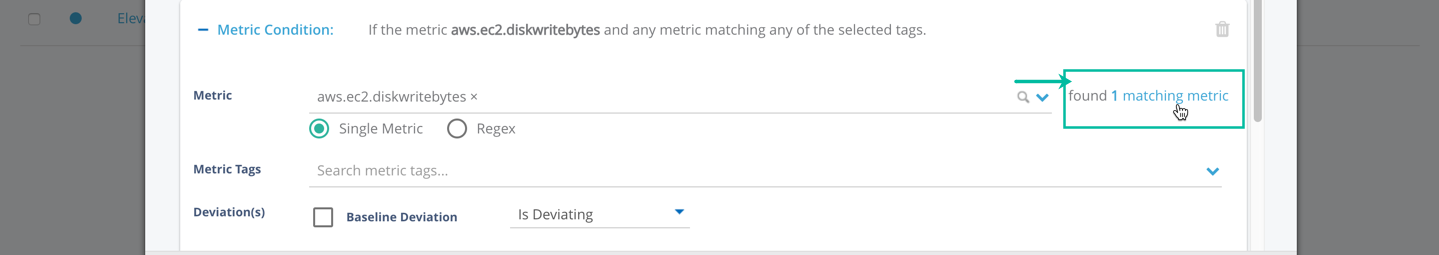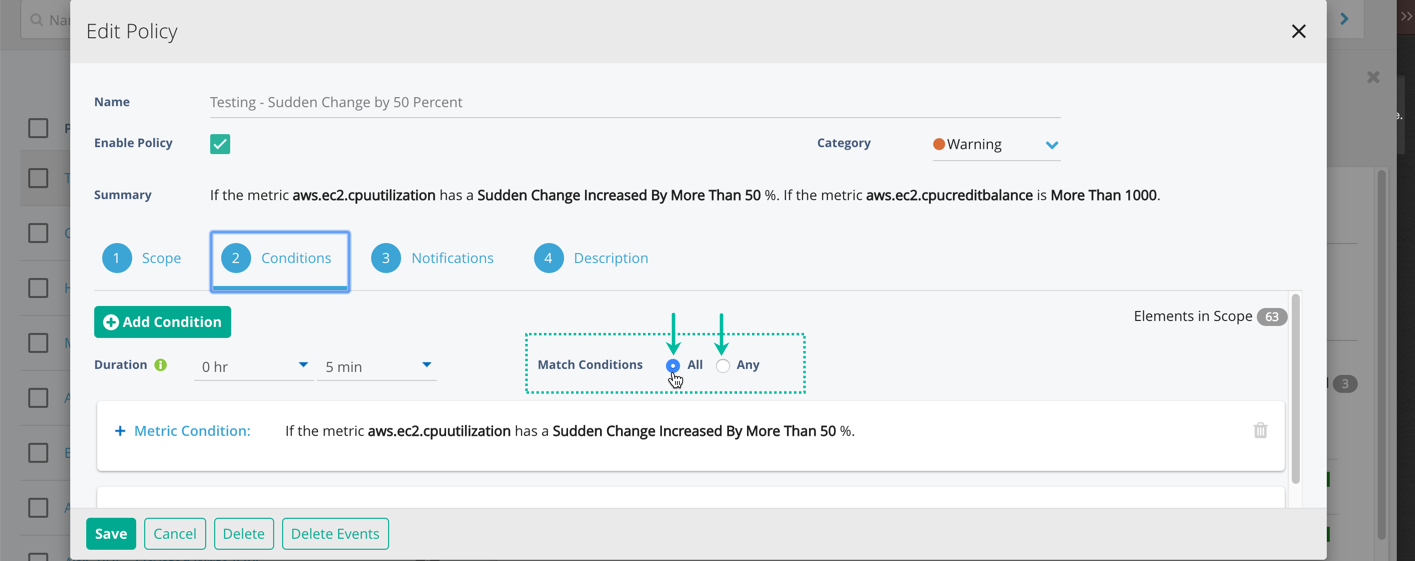# Create Conditions

When you are creating conditions, the Policy Editor counts the number of metrics that apply to the conditions you have set. To view those metrics, click on the link. This link opens the list of matching metrics in a new tab.## Create a Metric Condition

• To edit an existing policy, Navigate to Alerts > Show All Policies > Click `Policy Name` > Edit Policy > Conditions > Add Metric Conditions.
2. Select either the Single Metric or Regex radio button.
• Single Metric: Choose a metric from the Metric drop-down menu. This is the metric to which the condition will apply.
• Regex: Begin typing into the field. Stop typing when you’ve found the desired matching metrics. Each matching metric used by the policy is joined by an OR, meaning that only a single metric has to trigger the policy, not all of the matching metrics. Note that regular expressions are evaluated as case-insensitive, meaning uppercase and lowercase letters are treated the same. Keep this in mind if you have metrics with the same name but different casing.
3. Use the Metric Tags field to filter your condition (optional).
4. Select the deviations you want to track.
5. Click Save.

### Adding Multiple Metric Conditions to a Policy?

Use the Match Conditions feature to toggle between enforcing all conditions listed or just any one condition. See below:## Metric Condition Deviation Types

### Baseline Deviation

A Baseline Deviation test triggers an event and other optional notifications when the current value of a metric is above and/or below 4 standard deviations from its normal operating range. A Baseline Deviation test can also be used to trigger an event when the value of a metric is or is not deviating from its normal operating range. CloudWisdom determines the normal operating range of a metric based on the history of the actual values for that metric. The different Baseline Deviation tests are described below:

• Upper (Baseline) Deviation: The current value of a metric is greater than or equal to 4 standard deviations above its normal operating range.
• Lower (Baseline) Deviation: The current value of a metric is greater than or equal to 4 standard deviations below its normal operating range.
• Is Deviating: The current value of a metric is greater than or equal to 4 standard deviations above or below its normal operating range.
• Is Not Deviating: The current value of a metric is not deviating.

### Contextual Deviation

A Contextual Deviation test can be used to indicate when the value of a metric is above and/or below 4 standard deviations from its expected value. A Contextual Deviation test can also be used to indicate when a metric is deviating when it should not be, or is not deviating when it should be. CloudWisdom determines the expected value for a metric based on the actual values of other correlated metrics in the learned model. The different Contextual Deviation tests are described below:

• Upper (Contextual) Deviation: The current value of a metric is greater than or equal to 4 standard deviations above its expected value.
• Lower (Contextual) Deviation: The current value of a metric is greater than or equal to 4 standard deviations below its expected value.
• Is Deviating: The current value of a metric is greater than or equal to 4 standard deviations above or below its expected value.
• Is Not Deviating: The current value of a metric is not deviating.

### Static Threshold

A Static Threshold test is used to trigger an event and other optional actions when the value of a metric is more than, less than, equal to, or not equal to a specified level. The level for a Static Threshold test can be any real number; the unit of the level depends on the metric to which it is applied.

For example, you can use a Static Threshold test to execute an event when the current value for the metric “CPU Utilization” is greater than 95%.

### Sudden Change Deviation

A Sudden Change deviation test is used to indicate the difference between expected change and unexpected change on a certain metric. This is achieved by using historical data to predict future data intervals. The historical data used to determine the future interval is a sliding window of one hour that contextualizes future intervals.

The Analytics Engine uses the following steps to detect a sudden change:

1. Collects the last N+1 observed PT5M values, including the most recent value.
2. Applies a regression model to the N PT5M average values before the current one.
• If the regression model is a good fit, then use it to forecast a projected value of the metric.
3. Compares the projected regression model value with the actual observed value.
• This step determines whether the observed value is within the projected configurable range of confidence.
4. If observed value is within expected range, returns a result of NO CHANGE.
5. If observed value is not within expected range, compute percent change.

`Percent change = |(observed value - projected value)| / |projected value|`

## Metric Threshold

Metric thresholds are unchanging levels that are compared against another metric’s current value. A Metric Threshold test can be used to indicate when the value of the specified metric is more than, less than, equal to, or not equal to another metric.